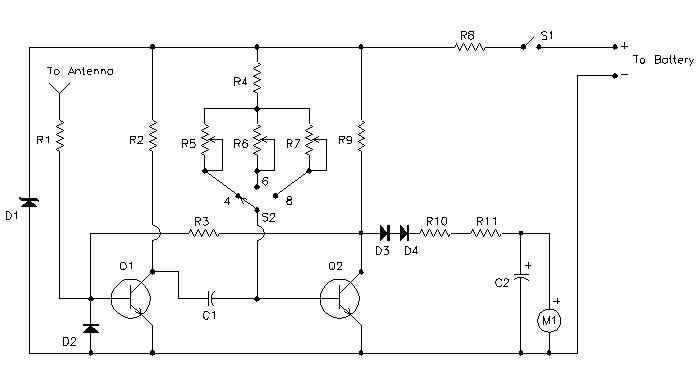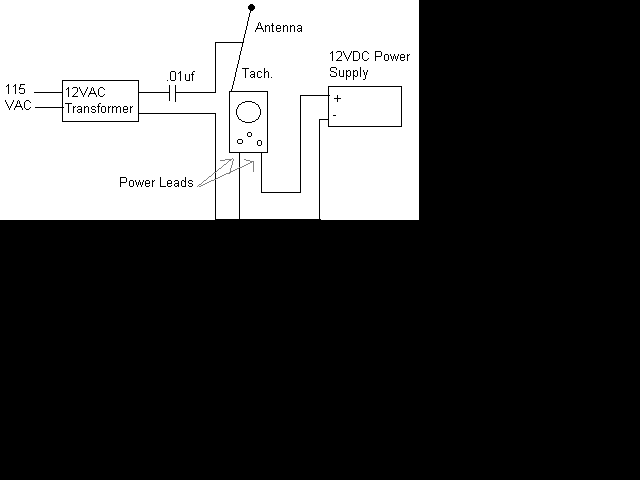### electronic universe

Nothing possible without electronics

.

## August 19, 2009

### Wireless auto tachometer

INTRODUCTION
Anyone performing their own automobile tune-ups knows how important it is to know your engines speed. With this tachometer, you can measure your engines speed without any connections or annoying timing lights.

circuit diagram### Notes

1. Calibrate the unit as folows:
1. Set up this circuit:2. Turn on the Tach and allow a few minutes for temperature stabilization.
3. Set S2 to 4 cylinders and adjust R5 for a meter indication of 180 (1800 rpm).
4. Set S2 to 6 cylinders and adjust R6 for a meter indication of 120 (1200 rpm).
5. Set S2 to 8 cylinders and adjust R7 for a meter indication of 90 (900 rpm).
2. To use the Tach, turn it on and let it sit for one minute to allow for temperature stabilization. Extend the antenna, select the right number of cylinders and hold the unit over the engine. If the reading is erratic or the needle jumps around, move the antenna closer to the ignition coil or spark plug wires.
The unit draws power from the car battery. If it is connected backwards, it will not work, but it won't be damaged.

parts required

 Part Total Qty. Description Substitutions C1 1 0.47uF Capacitor C2 1 47uF Electrolytic Capacitor D1 1 8V 1W Zener Diode D2, D3, D4 3 1N914 Diode M1 1 200uA Meter Q1, Q2 2 2N3391A Transistor R1, R2, R9 3 1K 1/2 W Resistor R3 1 47K 1/2 W Resistor R4 1 10K 1/2 W Resistor R5, R6 2 25K Trim Pot R7 1 10K Trim Pot R8 1 200 Ohm 2 W Resistor R10 1 15K 1/2 W Resistor R11 1 2.2K 1/2 W Resistor S1 1 SPST Togglae Switch S2 1 Three Position Single Pole Rotary Switch MISC 1 Telescoping Radio Antenna, Enclosure, Power Cable and Battery Connector

### Semi Log Graph Sheet

Semi Log Graph

They are mainly used for plotting very LARGE and very SMALL value in a same graph
Notice that the numbers along the x axis are evenly spaced, while along the y-axis, we have powers of 10 evenly spaced.

## August 16, 2009

### Conversion of Binary to Decimal

binary to decimal...

introduction:-

The binary (base two) numeral system has two possible values, often represented as 0 or 1, for each place-value. In contrast, the decimal (base ten) numeral system has ten possible values (0,1,2,3,4,5,6,7,8, or 9) for each place-value.
To avoid confusion while using different numeral systems, the base of each individual number may be specified by writing it as a subscript of the number. For example, the binary number 10011100 may be specified as "base two" by writing it as 100111002. The decimal number 156 may be written as 15610 and read as "one hundred fifty-six, base ten".

STEPS:-

1. For this example, let's convert the binary number 100110112 to decimal. List the powers of two from right to left. Start at 20, evaluating it as "1". Increment the exponent by one for each power. Stop when the amount of elements in the list is equal to the amount of digits in the binary number. The example number, 10011011, has eight digits, so the list, to eight elements, would look like this: 128, 64, 32, 16, 8, 4, 2, 1
2. Write the binary number below the list.
3.Here is this step written on paper using the example binary number, 10011011.
4. Draw lines, starting from the right, connecting each consecutive digit of the binary number to the power of two that is next in the list above it. Begin by drawing a line from the first digit of the binary number to the first power of two in the list above it. Then, draw a line from the second digit of the binary number to the second power of two in the list. Continue connecting each digit with its corresponding power of two.Here is this step written on paper using the example binary number, 10011011.
5. Move through each digit of the binary number. If the digit is a 1, write its corresponding power of two below the line, under the digit. If the digit is a 0, write a 0 below the line, under the digit.Here is this step written on paper using the example binary number, 10011011.
6. Add the numbers written below the line. The sum should be 155. This is the decimal equivalent of the binary number 10011011. Or, written with base subscripts: 100110112 = 15510

Here is this step written on paper using the example binary number, 10011011. The sum of the bottom row, 155, is its decimal equivalent. Or, written with base subscripts: 100110112 = 15510
7. Repetition of this method will result in memorization of the powers of two, which will allow you to skip step 1.

## Doubling method

Starting from zero, and working from left to right, double your number and add the next digit of the base two representation. For example to convert 1011001, we take the following steps.

1. 1|011001 0*2+1 = 1
2. 10|11001 1*2+0 = 2
3. 101|1001 2*2+1 = 5
4. 1011|001 5*2+1 = 11
5. 10110|01 11*2+0 = 22
6. 101100|1 22*2+0 = 44
7.1011001 44*2+1 = 89

## Tips

• Practice. Try converting the binary numbers 110100012, 110012, and 111100012. Respectively, their decimal equivalents are 20910, 2510, and 24110.
• The calculator that comes installed with Microsoft Windows can do this conversion for you, but as a programmer, you're better off with a good understanding of how the conversion works. The calculator's conversion options can be made visible by opening its "View" menu and selecting "Scientific". On Linux, you can use galculator.
• There is another way to convert from binary to decimal, which ignores the binary values of the columns. Take the left-most one digit and start there as "1." For each column to the right, double your subtotal and add the next digit. For example, "1011" would entail the following: "1," (double) 2, (add 0) 2, (double) 4, (add 1) 5, (double) 10, and finally (add 1) 11. This technique is very useful for converting large numbers in your head, as you only need to keep track of your sub-total (you are simply adding and doubling).

DOOR BELL FOR DEAF CLICK HERE

Electronics is the study and use of electrical that operate by controlling the flow of electrons or other electrically charged particles in devices such as thermionic valves. and semiconductors. The pure study of such devices is considered as a branch of physics, while the design and construction electronic circuits to solve practical problems is called electronic engineering.